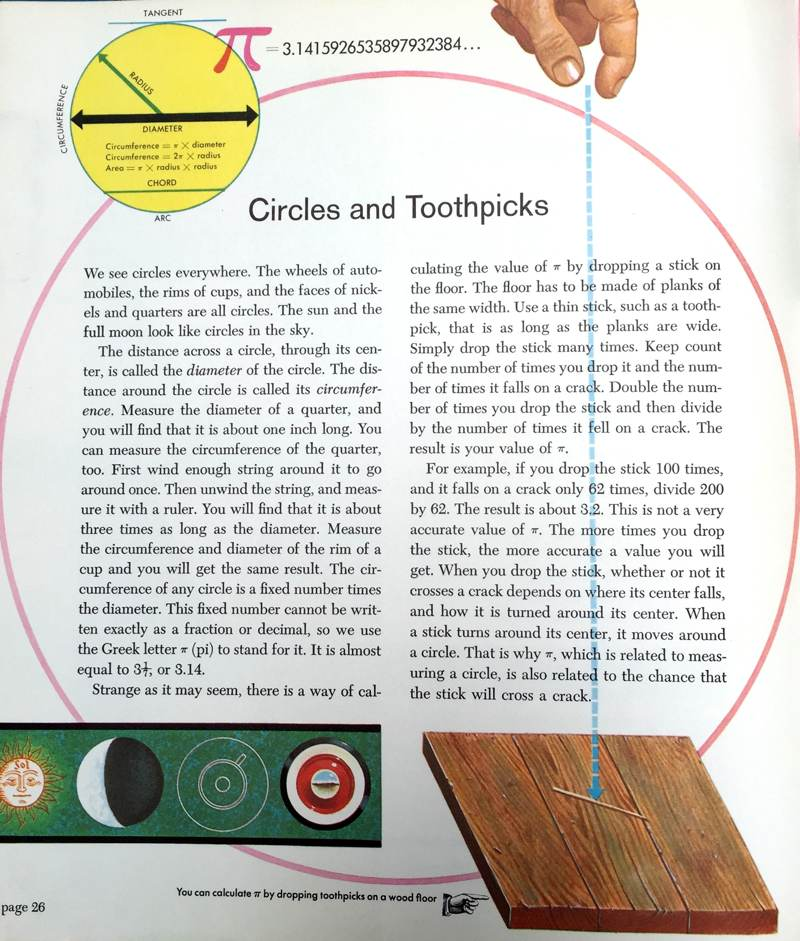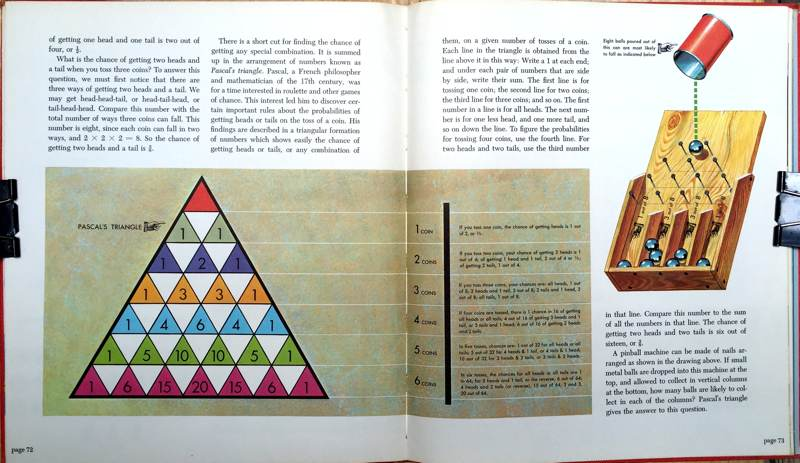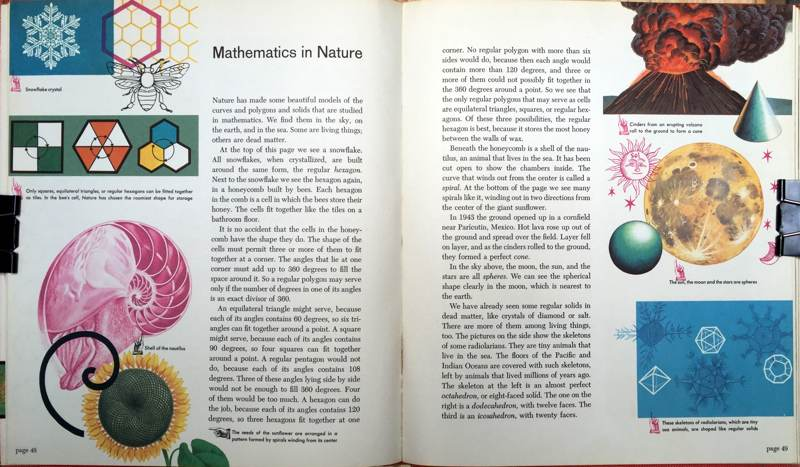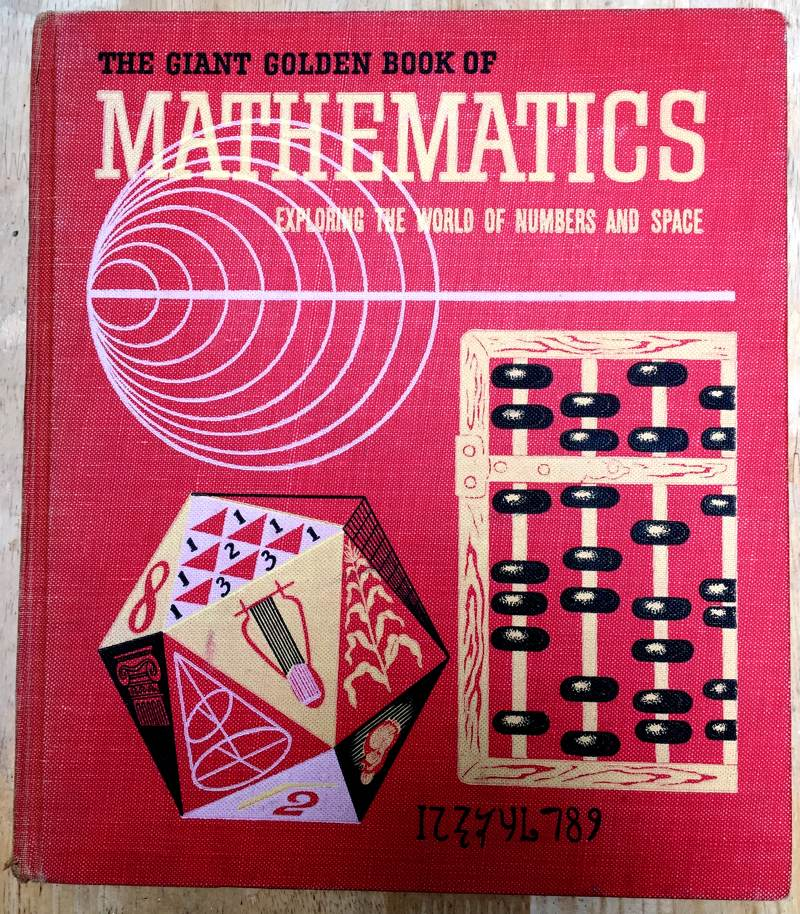# You can calculate Pi by dropping toothpicks on a wood floorIn honor of Pi Day (3/14/16 = 3.1416), here's a page from The Giant Golden Book of Mathematics (1958) that shows you how to calculate Pi by dropping toothpicks on a wood floor.

We see circles everywhere. The wheels of automobiles, the rims of cups, and the faces of nickels and quarters are all circles. The sun and the full moon look like circles in the sky.

The distance across a circle, through its center, is called the diameter of the circle. The distance around the circle is called its circumference. Measure the diameter of a quarter, and you will find that it is about one inch long. You can measure the circumference of the quarter, too. First wind enough string around it to go around once. Then unwind the string, and measure it with a ruler. You will find that it is about three times as long as the diameter. Measure the circumference and diameter of the rim of a cup and you will get the same result. The circumference of any circle is a fixed number times the diameter. This fixed number cannot be written exactly as a fraction or decimal, so we use the Greek letter pi to stand for it. It is almost equal to 3-1/7, or 3.14.

Strange as it may seem, there is a way of calculating the value of pi by dropping a stick on the floor. The floor has to be made of planks of the same width. Use a thin stick, such as a toothpick, that is as long as the planks are wide. Simply drop the stick many times. Keep count of the number of times you drop it and the number of times it falls on a crack. Double the number of times you drop the stick and then divide by the number of times it fell on a crack. The result is your value of pi.

For example, if you drop the stick 100 times, and it falls on a crack only 62 times, divide 200 by 62. The result is about 3.2. This is not a very accurate value of pi. The more times you drop the stick, the more accurate a value you will get. When you drop the stick, whether or not it crosses a crack depends on where its center falls, and how it is turned around its center. When a stick turns around its center, it moves around a circle. That is why pi, which is related to measuring a circle, is also related to the chance that the stick will cross a crack.

By the way, The Giant Golden Book of Mathematics is a large book filled with stunning illustrations. It's part of a series, which includes Birds, Biology, Dinosaurs, and oddly enough, Elves and Fairies. Here are a few sample pages:Here's a link to a PDF of The Giant Golden Book of Mathematics.